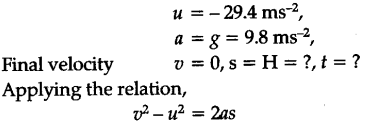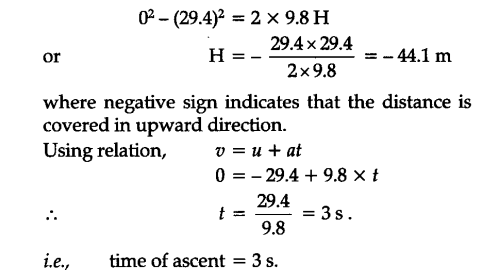# A player throws a ball upwards with an initial speed of 29.4 ms-1

A player throws a ball upwards with an initial speed of 29.4 ms-1.
(a) What is the direction of acceleration during the upward motion of the ball ?
(b) What are the velocity and acceleration of the ball at the highest point of its motion ?
© Choose the r = 0m and t = 0 s to be the location and time of the ball at its highest point, vertically downward direction to the positive direction of x-axis, and give the signs of position, velocity and acceleration of the ball during its upward and downward motion.’
(d) To what height does the ball rise and after how long does the ball return to the player’s hands ? (Take g = 9.8 ms-2 and neglect air resistance)

(a) As the ball is moving under the effect of gravity, the direction of acceleration due to gravity remain vertically downwards.
(b) If the ball is at the highest point of its motion, its velocity becomes zero and the acceleration is equal to the acceleration due to gravity = 9.8 ms-2 in vertically downward direction.
© If the highest point is chosen as the location for x = 0 and t = 0 and vertically downward direction to be the positive direction of x-axis.
For upward motion, sign of position is negative, sign of velocity is negative and the sign of acceleration is positive, i.e., v < 0, a > 0.
For downward motion, sign of position is positive, sign of velocity is positive and the sign of acceleration is also positive, i.e., v < 0, a > 0.
(d) Suppose, t = time taken by the ball to reach the highest point.
H = height of the highest point from the ground. During vertically upward motion of the ball,
. Initial velocity,It is also well known that when the object moves under the effect of gravity alone, the time of ascent is always equal to the time of descent.Total time after which the ball returns to the player’s hand = 2f = 2 x 3 = 6 s.